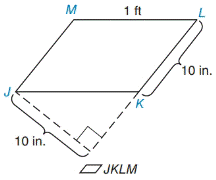Chapter 8.1, Problem 15E### Elementary Geometry for College St...

6th Edition
Daniel C. Alexander + 1 other
ISBN: 9781285195698

#### Solutions

Chapter
Section### Elementary Geometry for College St...

6th Edition
Daniel C. Alexander + 1 other
ISBN: 9781285195698
Textbook Problem
1 views

# In Exercises 9 to 18, find the areas of the figures shown or described.To determine

To find:

The area of the figure JKLM.

Explanation

1) Formula:

Area of the Parallelogram A=bh unit2.

Where, b is the base of the Parallelogram, and h is the height of the Parallelogram.

2) Calculation:

Given,

The given figure is a parallelogram JKLM with base b=10 in.,

Side s=1 ft=12 in.,

Let N be the point on the Parallelogram,

Where the LK is extended externally as LN which is perpendicular to JN that distance is 10 in

### Still sussing out bartleby?

Check out a sample textbook solution.

See a sample solution

#### The Solution to Your Study Problems

Bartleby provides explanations to thousands of textbook problems written by our experts, many with advanced degrees!

Get Started

#### Find more solutions based on key concepts